#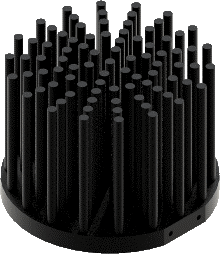### Why heat sink is important for LED lights?

LED, Lighting Emitting Diode, is the semiconductor component which can transfer the electric energy into light. However, in the process of power transfer, apart from emitting lights, there is lot of power will be converted into thermal energy therefore generating heat.

In general, based on current LED manufacture technology, regarding the LEDs with CRI value of 80-90 Ra, its efficacy will be about 30%-40%. In other words, there is only 30%-40% electric power transferred into light, the rest 60%-70% is transferred into heat. While for some special Ultra High CRI LEDs, such as YUJI High CRI LEDs, with CRI value of 95-98 Ra, this ratio of efficacy will be about 20% (BC series 100W LED), 80% electric power will be transferred into heat.

Emitting such high-level heat, LEDs would be damaged due to the over-heating without an effective cooling system. Technically, the over-heating of PN junction (Tj) will cause lumen output decay and even cause the death of LEDs. For example, Figure 1 is the relationship curve of YUJILEDS™ BC 135L COB (typical 9W) between case temperature and lumen output change.Figure 1: Case Temperature VS Relative Luminous output.

As we can see from the graph, when Tc rises, the luminous output will decay somewhat. When this temperature reaches at 105°C, namely the temperature of *Tj is above 125°C(Tj ≈ 1.2*TC), the luminous output of LEDs will be not good. When this decay runs up to 30%, according to the industrial standard, the LED will be recognized as defective product.

Therefore, for the sake of protecting LEDs performance, a good heat management is necessary.

### So, how to choose a good heat sink for your LEDs?

When designing an off-the-shelf light, matching a good heat sink is not easy. Unless the manufacturer of LEDs has already pointed out the specific recommendation, yet you have to make the calculation and choice by yourself.

Matching a suitable cooler, the key step is thermal calculation. Before making your choice of picking a good heat sink, what you need to do is the calculation of the required LED heat sink thermal resistance: *Rth. In this post, we will emphasize the method to do this calculation and to find a suitable cooler accordingly.

To start with, we need to know how the heat is transmitted from inside of LED die to the air.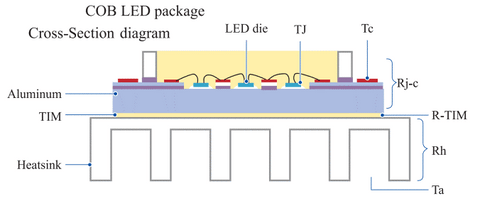Figure 2. COB LED package Cross-Section diagram

As can be seen from Figure 2, the procedure of heat transmission will be as follow.

1. The heat is generated from interior of LED die, from PN junction.
2. The heat transmits from LED die to the case of LED COB.
3. The heat transmits from the case to thermal interface material.
4. The heat transmits from thermal interface material to heat sink.
5. The heat transmits from heat sink to air.

After understanding this procedure, we are able to do the calculation and choice. The steps will be as follow.

• Step 1: Calculate the dissipated power: *Pd
• Step 2: Calculate the ambient temperature: *TA
• Step 3: Determine the ideal case temperature: *TC
• Step 4: Choose a good thermal interface material with good thermal resistance: *Rth.TIM
• Step 5: Calculate the required thermal resistance of heat sink: *Rth
• Step 6: Choose a good heat sink brand to purchase the ideal cooler

In this post, we will introduce the method of matching a good heat sink with an example of YUJILEDS™ 135L COB. Figure 3 is the test report.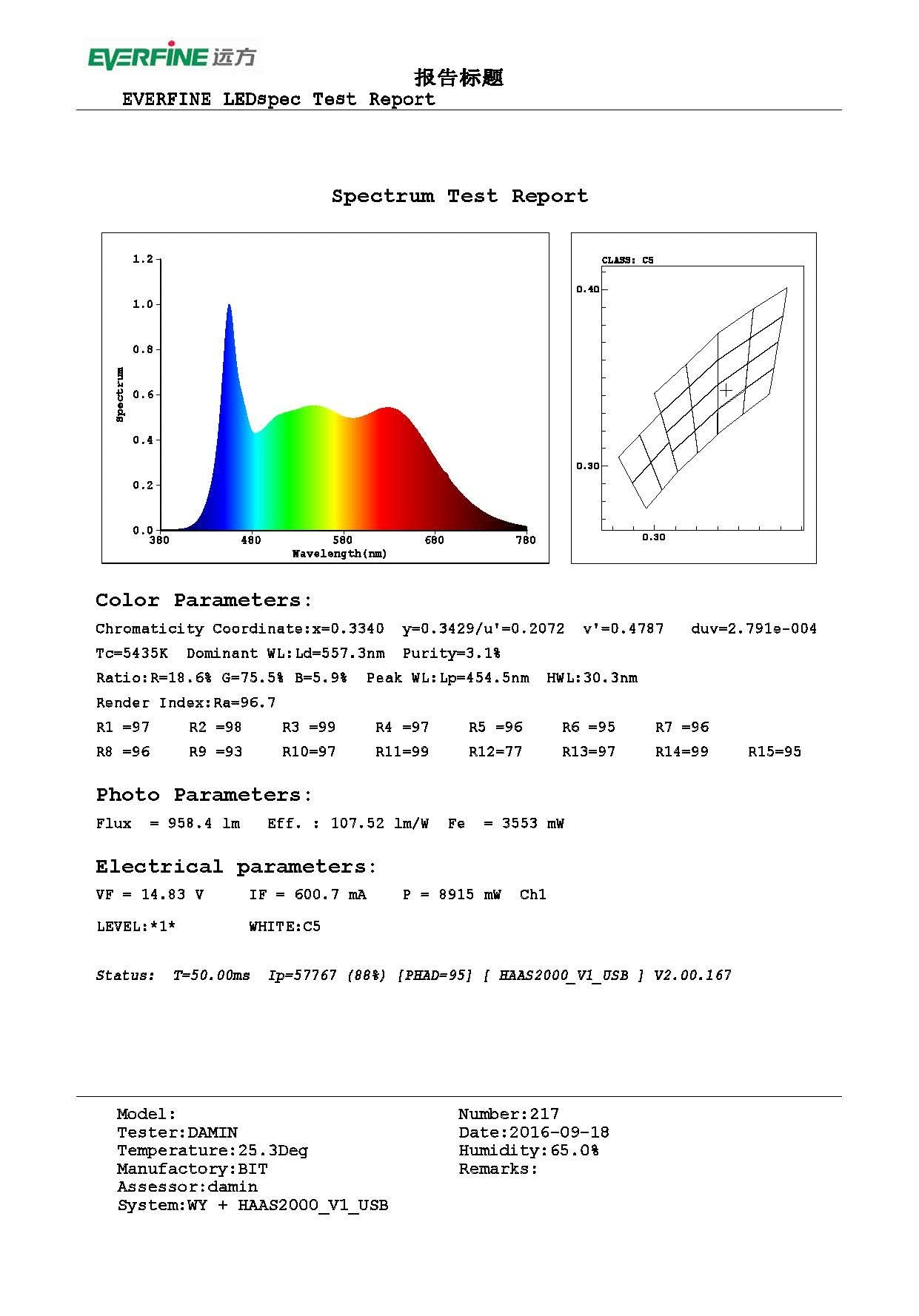Figure 3: the test report of YUJILEDS™ BC 135L COB

#### STEP 1: Calculate the dissipated power: *Pd

Dissipated Power, also namely thermal power, is the measurement of the level of energy changes into the heat form from electric energy. When a LED COB lit, it will emit light and heat. In general, we call the power changes into light as “light watts”, and the power changes into heat as “heat watts”.

As shown in the test report in Figure 3, the number of light watts is Fe: 3553mW = 3.55W and total electric power is Pe = If*Vf = 15*600 ≈ 9000 mW.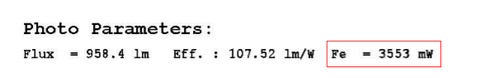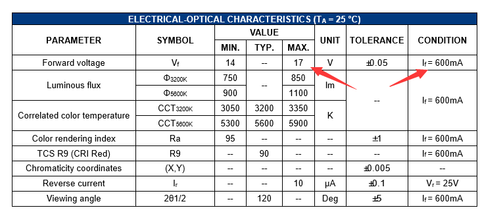Therefore, in estimate, we can consider that 3.55W electric power changes into light and the rest 5.45W changes into heat, which is dissipated power Pd, should be dissipated by a suitable cooler. In other words, the efficacy of this 9W LED COB is about 40%. The formula will be as follow.

Pd=Fe*(1-Eff)=9*(1-39.5%)≈5.45W

If you want to know the dissipated power of an LED, you ought to get it, or at least the efficacy, from its manufacturer.

#### STEP 2: Calculate the ambient temperature: *TA

This step is simple. In general, you can get the number of TA from datasheet of the LED manufacturer. Generally the marked TA in a typical LED datasheet is 25°C.

#### STEP 3: Determine the ideal case temperature: *Tc

The value of case temperature limit is also the one we can find from datasheet.

Figure 4 is from the datasheet of YUJILEDS™ BC 135L COB.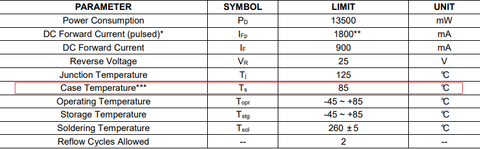Figure 4: the parameter limitations of YUJILEDS™ BC 135L COB

Then we can easily define a best working case temperature lower than 85℃, let’s say 60°C for instance as the best condition for your working LED COB.

#### STEP 4: Choose a good thermal interface material with good thermal resistance: *Rth.TIM

The choice of thermal interface material is crucial. It will impact the working performance of the whole cooling system. For easy choice, our recommendation is to find a good material with best thermal resistance, such as 0.1 – 0.2°C/W. In this case, we choose a thermal interface material with Rth.TIM = 0.2°C/W.

#### STEP 5: Calculate the required thermal resistance of heat sink: *Rth

After we have the numeral result of *Pd，*TA，*TC，*Rth.TIM, *Dt, now we are able to calculate the thermal resistance of a good heat sink. The formula is as follow.

Rth=dT/Pd-Rth.TIM=(50-25)/5.45-0.2=4.38°C/W

In terms of the value of Rth, the lower it is, the better its performance will be.

#### STEP 6: Choose a good heat sink brand to purchase the ideal cooler

The heatsink brands we recommend are as follow.

1. MechaTronix: https://www.led-heatsink.com
2. MingFa: https://www.mingfatech.com
3. Cooliance: http://www.cooliance.com/

*Pd: is heat dissipated at the junction and conducting through the LED Modules.

*Tj: is the temperature at the junction of the device.

*Tc: is the case temperature.

*dT: is the temperature at the point where the heat sink is attached to the substrate.

*TA: is the ambient air temperature.

*Rth.TIM: is the thermal resistance between the case and the heat sink (TIM resistance).

*Rth: is the thermal resistance of the heat sink.